Diffie Hellman Key Exchange Algorithm – Asymmetric Key Cryptography !!

The Diffie Hellman algorithm was widely known as the Key exchange algorithm or key agreement algorithm developed by Whitfield Diffie and Martin Hellman in 1976. Diffie Hellman algorithm is used to generate same (symmetric) private cryptographic key at the sender as well as receiver end so that there is no need to transfer this key from sender to receiver.

Remember that the Diffie Hellman algorithm is used only for key agreement, not for encryption or decryption of message. If the sender and receiver want to communicate with each other they first agree on the same key generated by Diffie Hellman Algorithm later on they can use this key for encryption or decryption. Let us start with the algorithm.Without wasting any further time, let’s start understanding the steps for executing the Diffie Hellman Key Exchange Algorithm process.

Steps of Diffie Hellman Algorithm !!

The main idea behind this Diffie Hellman Key Exchange process basically lies in the idea of splitting a secret key into several parts . Out of several parts , one part will be known to public (not confidential) , the other parts will be then added in parts to make a full key similar to that sent by the sender initially .

1. The first step is that if Ramesh wants to communicate with Suresh they must agree on two large prime numbers p and q.

2. Ramesh selects another secret large random integer number a, and calculate R such that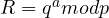3. Ramesh sends this ‘R‘ to Suresh.

4. Suresh independently selects another secret large random integer number b, and calculate S such that.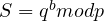5. Suresh sends the number S to Ramesh.

6. Now Ramesh is calculating his secret key by using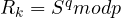7. Suresh is calculating his secret key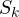by using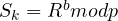8. If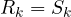then Ramesh and Suresh can agree for future communication called as key agreement algorithm.

9. We have= K hence proved. (K is called symmetric key).

For example:

1. Ramesh and Suresh agree on two large prime numbers say p = 17 and q = 7.

Note : These p and q numbers can be declared to public . These two numbers need not be confidential. They can be sent through the Internet they can be public

2. Ramesh selects another secret large random number 5 i.e. a = 5 and calculate R such that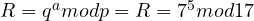= 11 = (7 x 7 x 7 x7 x 7) mod 17 = 11

3. Ramesh sends number R to Suresh.

4. Suresh selects another secret large random number 3. i.e. b = 3 and calculate S such that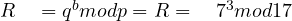= 3 = (7 x 7 x 7) mod 17 = 3

5. Suresh sends number S to Ramesh.

6. Ramesh now calculates its secret key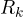as follows :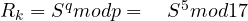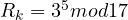= 5 = (3x 3 x 3 x 3 x 3) mod 17 = 5.

7. Suresh is calculating his secret keyas follows :=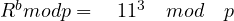= 5

8. Ifthen Ramesh and Suresh can agree for future communication.

9. We know that if== K = 5. Hence proved.

As seen above finally the plain text got retrieved by the receiver side by using the Diffie Hellman Key Exchange cryptography algorithm . In this cryptographic method , two parties create a symmetric session key to exchange data without having to remember or store the key for future use. They do not have to meet to agree on the key; it can be done through the Internet.

Note : Diffie Hellman Key Exchange is not exactly an encryption and decryption process , rather it is used as a key exchange method/agreement

Attacks In Diffie Hellman Key Exchange Algorithm !!

Diffie Hellman key exchange algorithm creates one discrepancy called a man-in-the-middle attack. Man-in-the-middle attack also called a bucket brigade attack.

However, the protocol does have a weakness. Any intruder does not have to find the value of p and q (as above) to attack the protocol. She (intruder) can fool Ramesh and Suresh by creating two keys i.e. one between herself and Ramesh and another between herself and Suresh.

Here the sender and receiver not aware that the integrity (after sender sends it and before it reaches to intended recipients’ original contents gets modified) of the message is lost. Because of interception sender and receiver not aware that third person listening to their communication.

Hope you really liked this post about the Diffie Hellman Key Exchange process . Stay tuned for more stuff in this series .